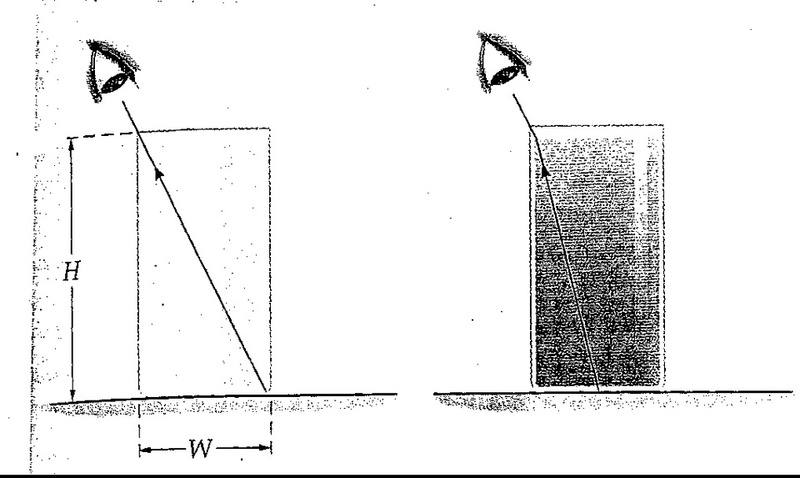# Using snell's law, observations thru a glass of water & glass of air

imatreyu

## Homework Statement

The observer in the figure shown below is positioned so thatthe far edge of the empty glass is just visible. When theglass is filled with water, the center of the bottom of the glassis just visible to the observer. Calculate the height H of theglass if its width W=4 cm.snell's law

## The Attempt at a Solution

sin theta = 2r/root(4r^2 + h^2) -AIR
sin phi = r/ root(r^2+h^2) -WATER

plug into snell's law:

n1sintheta = n2sinphi

(1) 2r/root(4r^2 + h^2) = (1.333) r/ root(r^2+h^2)

And then from there I solve for h. . . i cross multiply to eliminate the denominators and then square both sides to get rid of the square root signs.

So then. .

h^2 = 5.6 cm
h= 2.366 cm.

Please help! I don't know what I'm doing wrong, but the answer doesn't seem reasonable at all. :( It's totally insane. . .

hi imatreyu!(have a square-root: √ and try using the X2 icon just above the Reply box)
that's a bit long-winded, but i get the same answer, 2√(7/5)